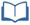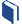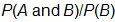# Prize Winner, Part 1

Estimated Time: 90 minutes

###Lesson Objectives:

• The students will understand and find independent and dependent conditional probabilities.
• The students will recognize, explain and interpret conditional probabilities given everyday language and everyday situations.

###Resources for this lesson:

Before you begin, download your Algebra II Journalfor Prize Winner, Part 1. You will be completing activities in the journal throughout this lesson.

Visit this section on each page to access information about key terms and other resources to help facilitate the lesson.

> Glossary> Calculator Resources> Teacher Resources: Instructional NotesKey Common Core State Standards:

S.CP.2: Understand that two events A and B are independent if the probability of A and B occurring together is the product of their probabilities, and use this characterization to determine if they are independent.

S.CP.3: Understand the conditional probability of A given B as, and interpret independence of A and B as saying that the conditional probability of A given B is the same as the probability of A, and the conditional probability of B given A is the same as the probability of B.

S.CP.5: Recognize and explain the concepts of conditional probability and independence in everyday language and everyday situations. For example, compare the chance of having lung cancer if you are a smoker with the chance of being a smoker if you have lung cancer.

S.CP.6: Find the conditional probability of A given B as the fraction of B’s outcomes that also belong to A, and interpret the answer in terms of the model.

Supporting Common Core State Standards:

7.SP.C.8: Find probabilities of compound events using organized lists, tables, tree diagrams, and simulation.

7.SP.C.8a: Understand that, just as with simple events, the probability of a compound event is the fraction of outcomes in the sample space for which the compound event occurs.

7.SP.C.8b: Represent sample spaces for compound events using methods such as organized lists, tables and tree diagrams. For an event described in everyday language (e.g., “rolling double sixes”), identify the outcomes in the sample space which compose the event.

S.CP.1: Describe events as subsets of a sample space (the set of outcomes) using characteristics (or categories) of the outcomes, or as unions, intersections, or complements of other events (“or”, “and”, “not”).

Standards for Mathematical Practices:

1. Make sense of problems and persevere in solving them.
2. Reason abstractly and quantitatively.
3. Construct viable arguments and critique the reasoning of others.
4. Model with mathematics.
5. Use appropriate tools strategically.
6. Attend to precision.
7. Look for and make use of structure.
8. Look for and express regularity in repeated reasoning.

Next >